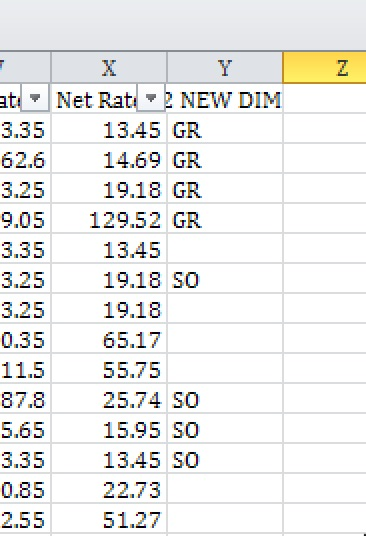# VBA add condition to if

I need an additional line that will say,
if the values in column A do NOT match with "Enter Data" G6, G7 or G8,
just put the existing value from Column A into  .Cells(lngRow, 25)

Can someone help?

``````Sub UpdateReducedData()
Dim lngLastRow As Long
Dim lngRow As Long

With Sheets("Reduced_Data")
lngLastRow = .range("A1048576").End(xlUp).Row
For lngRow = 2 To lngLastRow
If .Cells(lngRow, 1) = Sheets("Enter Data").range("D6") Then
.Cells(lngRow, 25) = Sheets("Enter Data").range("G6")
End If
If .Cells(lngRow, 1) = Sheets("Enter Data").range("D7") Then
.Cells(lngRow, 25) = Sheets("Enter Data").range("G7")
End If
If .Cells(lngRow, 1) = Sheets("Enter Data").range("D8") Then
.Cells(lngRow, 25) = Sheets("Enter Data").range("G8")
End If
Next

End With
End Sub
``````
###### Who is Participating?
I wear a lot of hats...

"The solutions and answers provided on Experts Exchange have been extremely helpful to me over the last few years. I wear a lot of hats - Developer, Database Administrator, Help Desk, etc., so I know a lot of things but not a lot about one thing. Experts Exchange gives me answers from people who do know a lot about one thing, in a easy to use platform." -Todd S.

Older than dirtCommented:
``````Sub UpdateReducedData()
Dim lngLastRow As Long
Dim lngRow As Long
Dim bFound As Boolean

With Sheets("Reduced_Data")
lngLastRow = .Range("A1048576").End(xlUp).Row
For lngRow = 2 To lngLastRow
bFound = False
If .Cells(lngRow, 1) = Sheets("Enter Data").Range("D6") Then
.Cells(lngRow, 25) = Sheets("Enter Data").Range("G6")
bFound = True
End If
If .Cells(lngRow, 1) = Sheets("Enter Data").Range("D7") Then
.Cells(lngRow, 25) = Sheets("Enter Data").Range("G7")
bFound = True
End If
If .Cells(lngRow, 1) = Sheets("Enter Data").Range("D8") Then
.Cells(lngRow, 25) = Sheets("Enter Data").Range("G8")
bFound = True
End If
Next
If Not bFound Then
.Cells(lngRow, 25) = .Cells(lngRow, 1)
End If
End With

End Sub
``````
Author Commented:
@Martin, it does not seem to work for me.
I entered into the project and attached.
Can you take a look?
Sub UpdateReducedData()
Rerate-v5.2.xlsm
Older than dirtCommented:
What do you mean when you say it's "not working"? It places these values in column "Y".Author Commented:
Sorry, I wasn't clear.
The blanks (that didn't match to Enter Data values), should be the corresponding value in Column A.
There should either be the replaced values, or the same value as Column A.
Older than dirtCommented:
Sorry.

``````Sub UpdateReducedData()
Dim lngLastRow As Long
Dim lngRow As Long
Dim bFound As Boolean

With Sheets("Reduced_Data")
lngLastRow = .Range("A1048576").End(xlUp).Row
For lngRow = 2 To lngLastRow
bFound = False
If .Cells(lngRow, 1) = Sheets("Enter Data").Range("D6") Then
.Cells(lngRow, 25) = Sheets("Enter Data").Range("G6")
bFound = True
End If
If .Cells(lngRow, 1) = Sheets("Enter Data").Range("D7") Then
.Cells(lngRow, 25) = Sheets("Enter Data").Range("G7")
bFound = True
End If
If .Cells(lngRow, 1) = Sheets("Enter Data").Range("D8") Then
.Cells(lngRow, 25) = Sheets("Enter Data").Range("G8")
bFound = True
End If
If Not bFound Then
.Cells(lngRow, 25) = .Cells(lngRow, 1)
End If
Next
End With

End Sub
``````

Experts Exchange Solution brought to you by

Your issues matter to us.

Facing a tech roadblock? Get the help and guidance you need from experienced professionals who care. Ask your question anytime, anywhere, with no hassle.

Author Commented:
No apology. Thanks so much!
###### It's more than this solution.Get answers and train to solve all your tech problems - anytime, anywhere.Try it for free Edge Out The Competitionfor your dream job with proven skills and certifications.Get started today Stand Outas the employee with proven skills.Start learning today for free Move Your Career Forwardwith certification training in the latest technologies.Start your trial today
Microsoft Excel

From novice to tech pro — start learning today.